# Selina Solutions Concise Maths Class 7 Chapter 3: Fraction (Including Problems) Exercise 3D

Selina Solutions Concise Maths Class 7 Chapter 3 Fraction (Including Problems) Exercise 3D provides students with the idea of using brackets and their removal, based on the latest syllabus. The solutions are prepared by teachers having knowledge about the concepts covered in the exercise. PDF of solutions has notes of important formulas and shortcut tricks to boost the exam preparation of students. It is the best study material and reference guide for the students to revise the concepts before the exam. Selina Solutions Concise Maths Class 7 Chapter 3 Fraction (Including Problems) Exercise 3D, PDF links are available below for free download.

## Selina Solutions Concise Maths Class 7 Chapter 3: Fraction (Including Problems) Exercise 3D Download PDF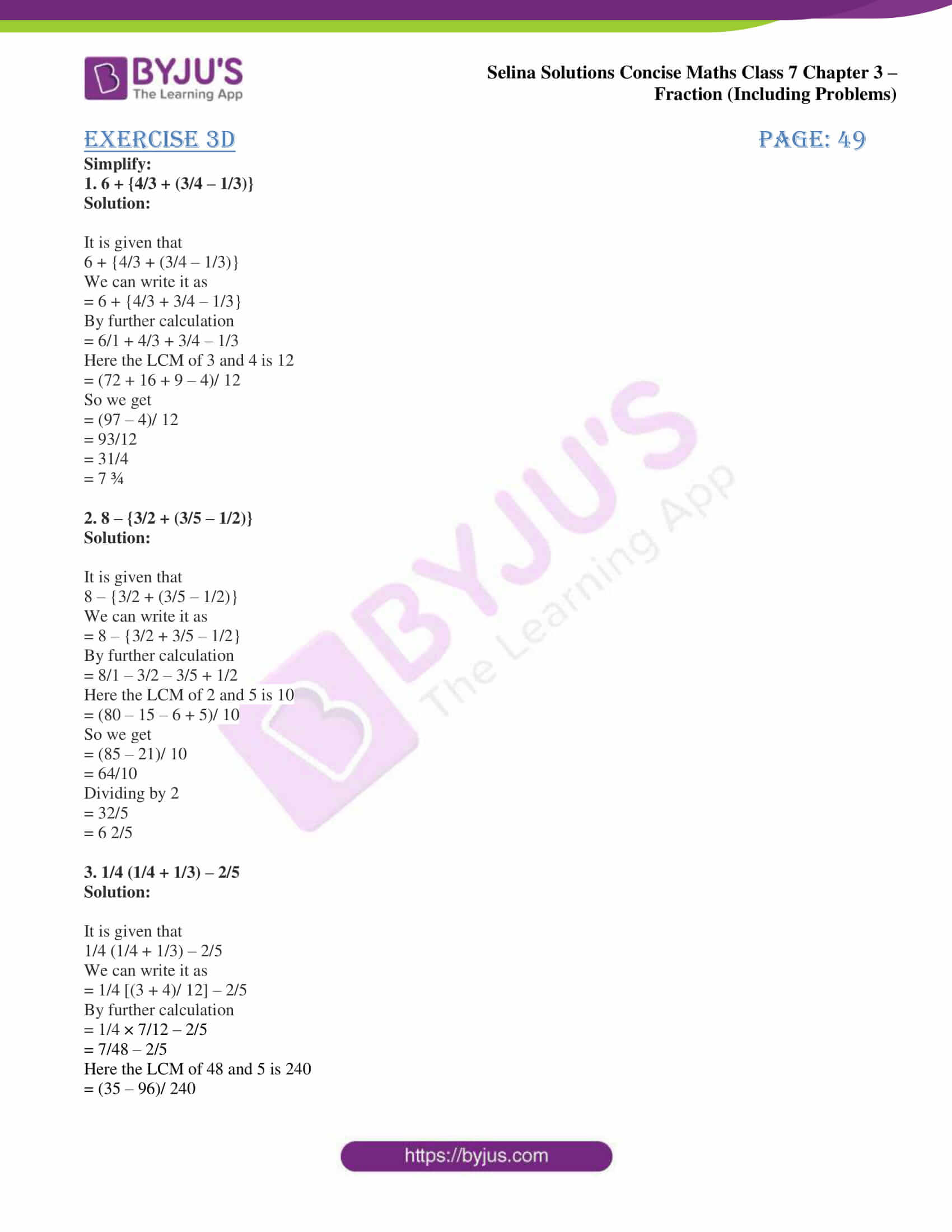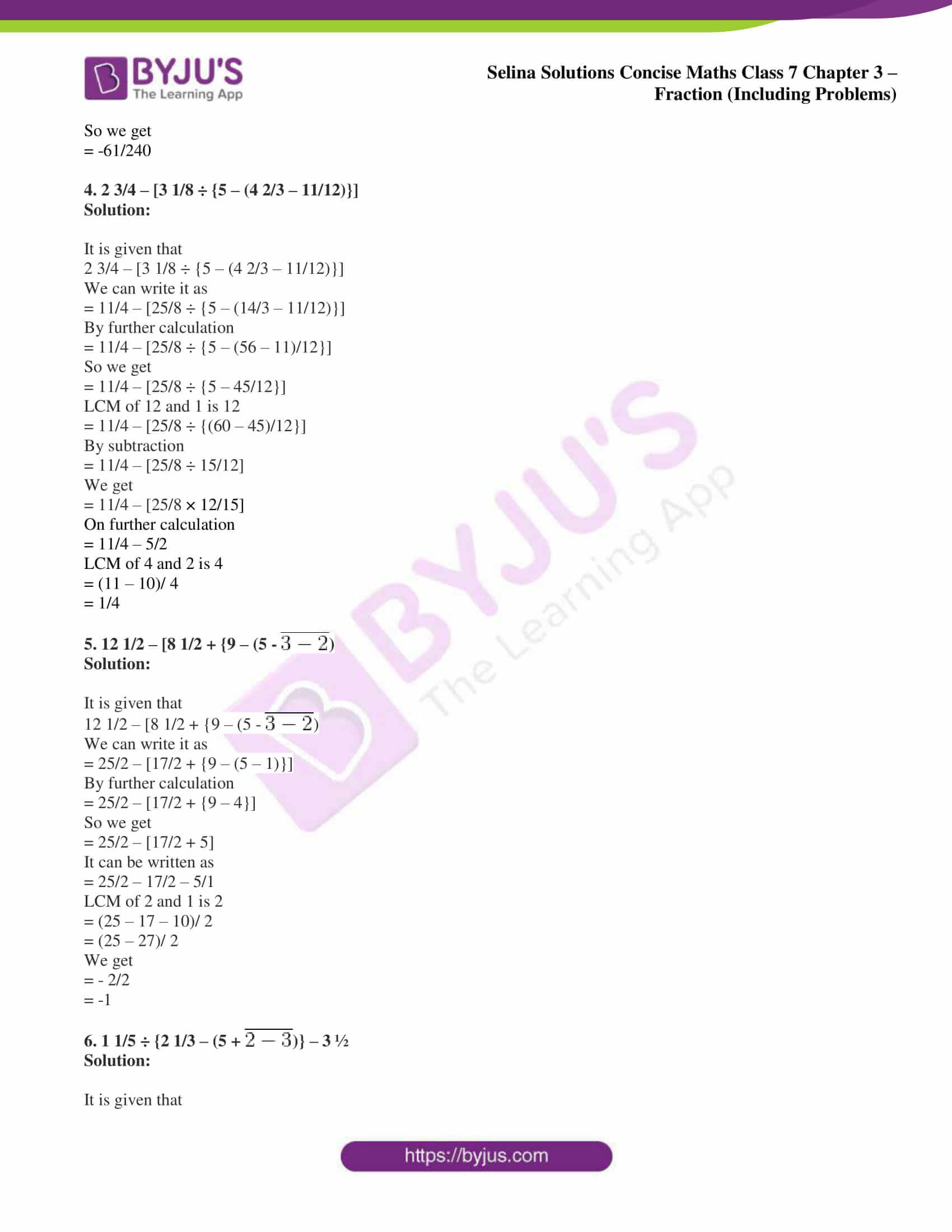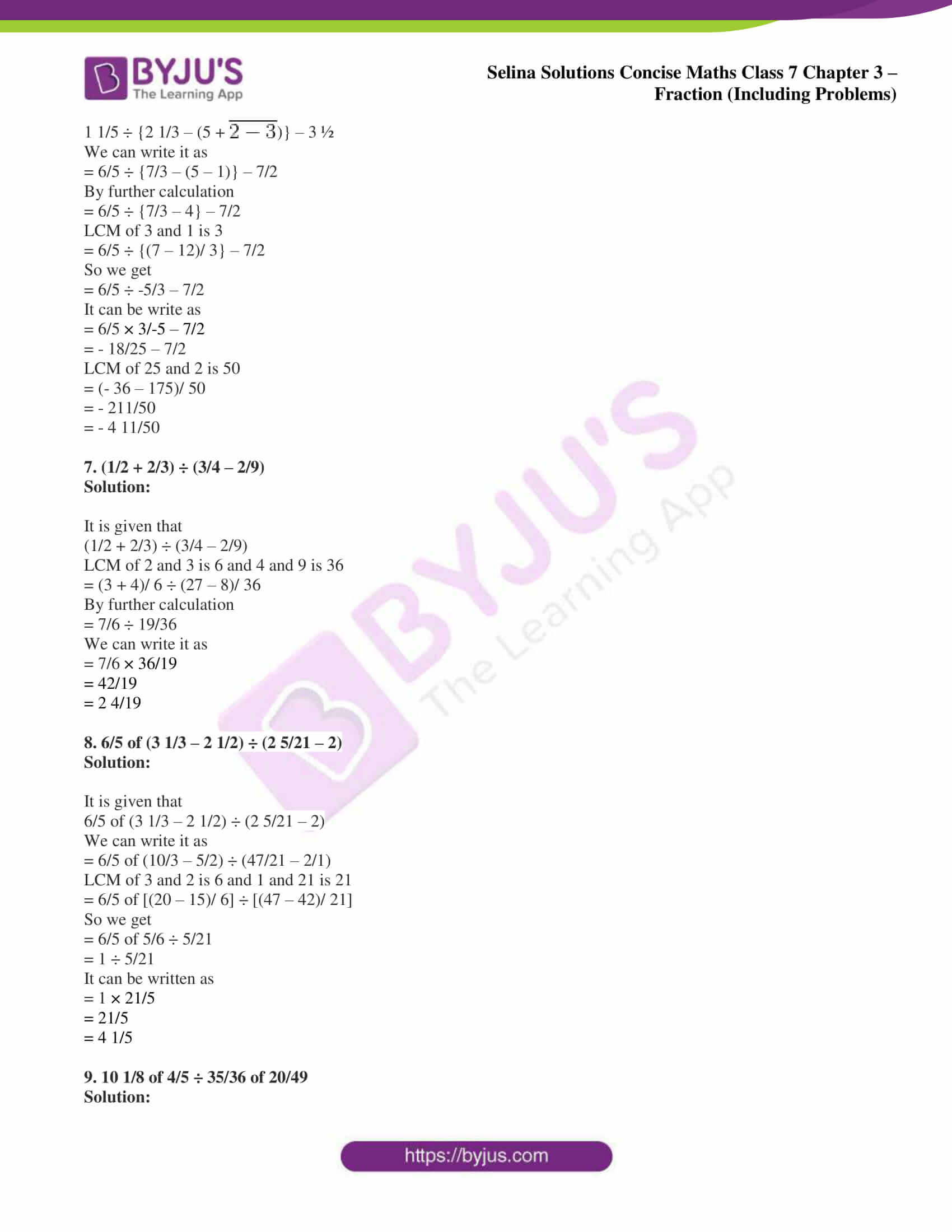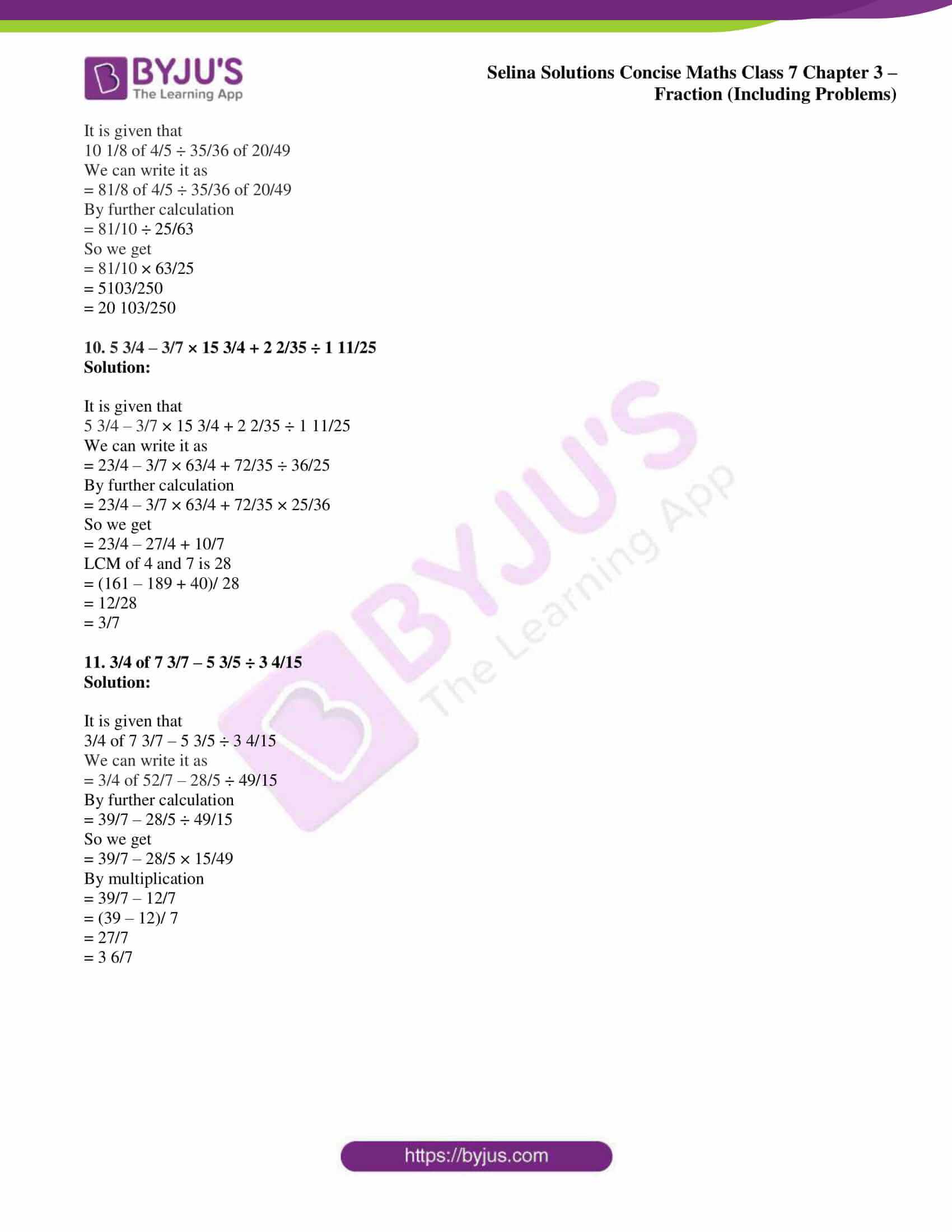### Access Selina Solutions Concise Maths Class 7 Chapter 3: Fraction (Including Problems) Exercise 3D

Simplify:

1. 6 + {4/3 + (3/4 – 1/3)}

Solution:

It is given that

6 + {4/3 + (3/4 – 1/3)}

We can write it as

= 6 + {4/3 + 3/4 – 1/3}

By further calculation

= 6/1 + 4/3 + 3/4 – 1/3

Here the LCM of 3 and 4 is 12

= (72 + 16 + 9 – 4)/ 12

So we get

= (97 – 4)/ 12

= 93/12

= 31/4

= 7 ¾

2. 8 – {3/2 + (3/5 – 1/2)}

Solution:

It is given that

8 – {3/2 + (3/5 – 1/2)}

We can write it as

= 8 – {3/2 + 3/5 – 1/2}

By further calculation

= 8/1 – 3/2 – 3/5 + 1/2

Here the LCM of 2 and 5 is 10

= (80 – 15 – 6 + 5)/ 10

So we get

= (85 – 21)/ 10

= 64/10

Dividing by 2

= 32/5

= 6 2/5

3. 1/4 (1/4 + 1/3) – 2/5

Solution:

It is given that

1/4 (1/4 + 1/3) – 2/5

We can write it as

= 1/4 [(3 + 4)/ 12] – 2/5

By further calculation

= 1/4 × 7/12 – 2/5

= 7/48 – 2/5

Here the LCM of 48 and 5 is 240

= (35 – 96)/ 240

So we get

= -61/240

4. 2 3/4 – [3 1/8 ÷ {5 – (4 2/3 – 11/12)}]

Solution:

It is given that

2 3/4 – [3 1/8 ÷ {5 – (4 2/3 – 11/12)}]

We can write it as

= 11/4 – [25/8 ÷ {5 – (14/3 – 11/12)}]

By further calculation

= 11/4 – [25/8 ÷ {5 – (56 – 11)/12}]

So we get

= 11/4 – [25/8 ÷ {5 – 45/12}]

LCM of 12 and 1 is 12

= 11/4 – [25/8 ÷ {(60 – 45)/12}]

By subtraction

= 11/4 – [25/8 ÷ 15/12]

We get

= 11/4 – [25/8 × 12/15]

On further calculation

= 11/4 – 5/2

LCM of 4 and 2 is 4

= (11 – 10)/ 4

= 1/4

5. 12 1/2 – [8 1/2 + {9 – (5 –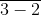)

Solution:

It is given that

12 1/2 – [8 1/2 + {9 – (5 –)

We can write it as

= 25/2 – [17/2 + {9 – (5 – 1)}]

By further calculation

= 25/2 – [17/2 + {9 – 4}]

So we get

= 25/2 – [17/2 + 5]

It can be written as

= 25/2 – 17/2 – 5/1

LCM of 2 and 1 is 2

= (25 – 17 – 10)/ 2

= (25 – 27)/ 2

We get

= – 2/2

= -1

6. 1 1/5 ÷ {2 1/3 – (5 +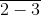)} – 3 ½

Solution:

It is given that

1 1/5 ÷ {2 1/3 – (5 +)} – 3 ½

We can write it as

= 6/5 ÷ {7/3 – (5 – 1)} – 7/2

By further calculation

= 6/5 ÷ {7/3 – 4} – 7/2

LCM of 3 and 1 is 3

= 6/5 ÷ {(7 – 12)/ 3} – 7/2

So we get

= 6/5 ÷ -5/3 – 7/2

It can be write as

= 6/5 × 3/-5 – 7/2

= – 18/25 – 7/2

LCM of 25 and 2 is 50

= (- 36 – 175)/ 50

= – 211/50

= – 4 11/50

7. (1/2 + 2/3) ÷ (3/4 – 2/9)

Solution:

It is given that

(1/2 + 2/3) ÷ (3/4 – 2/9)

LCM of 2 and 3 is 6 and 4 and 9 is 36

= (3 + 4)/ 6 ÷ (27 – 8)/ 36

By further calculation

= 7/6 ÷ 19/36

We can write it as

= 7/6 × 36/19

= 42/19

= 2 4/19

8. 6/5 of (3 1/3 – 2 1/2) ÷ (2 5/21 – 2)

Solution:

It is given that

6/5 of (3 1/3 – 2 1/2) ÷ (2 5/21 – 2)

We can write it as

= 6/5 of (10/3 – 5/2) ÷ (47/21 – 2/1)

LCM of 3 and 2 is 6 and 1 and 21 is 21

= 6/5 of [(20 – 15)/ 6] ÷ [(47 – 42)/ 21]

So we get

= 6/5 of 5/6 ÷ 5/21

= 1 ÷ 5/21

It can be written as

= 1 × 21/5

= 21/5

= 4 1/5

9. 10 1/8 of 4/5 ÷ 35/36 of 20/49

Solution:

It is given that

10 1/8 of 4/5 ÷ 35/36 of 20/49

We can write it as

= 81/8 of 4/5 ÷ 35/36 of 20/49

By further calculation

= 81/10 ÷ 25/63

So we get

= 81/10 × 63/25

= 5103/250

= 20 103/250

10. 5 3/4 – 3/7 × 15 3/4 + 2 2/35 ÷ 1 11/25

Solution:

It is given that

5 3/4 – 3/7 × 15 3/4 + 2 2/35 ÷ 1 11/25

We can write it as

= 23/4 – 3/7 × 63/4 + 72/35 ÷ 36/25

By further calculation

= 23/4 – 3/7 × 63/4 + 72/35 × 25/36

So we get

= 23/4 – 27/4 + 10/7

LCM of 4 and 7 is 28

= (161 – 189 + 40)/ 28

= 12/28

= 3/7

11. 3/4 of 7 3/7 – 5 3/5 ÷ 3 4/15

Solution:

It is given that

3/4 of 7 3/7 – 5 3/5 ÷ 3 4/15

We can write it as

= 3/4 of 52/7 – 28/5 ÷ 49/15

By further calculation

= 39/7 – 28/5 ÷ 49/15

So we get

= 39/7 – 28/5 × 15/49

By multiplication

= 39/7 – 12/7

= (39 – 12)/ 7

= 27/7

= 3 6/7